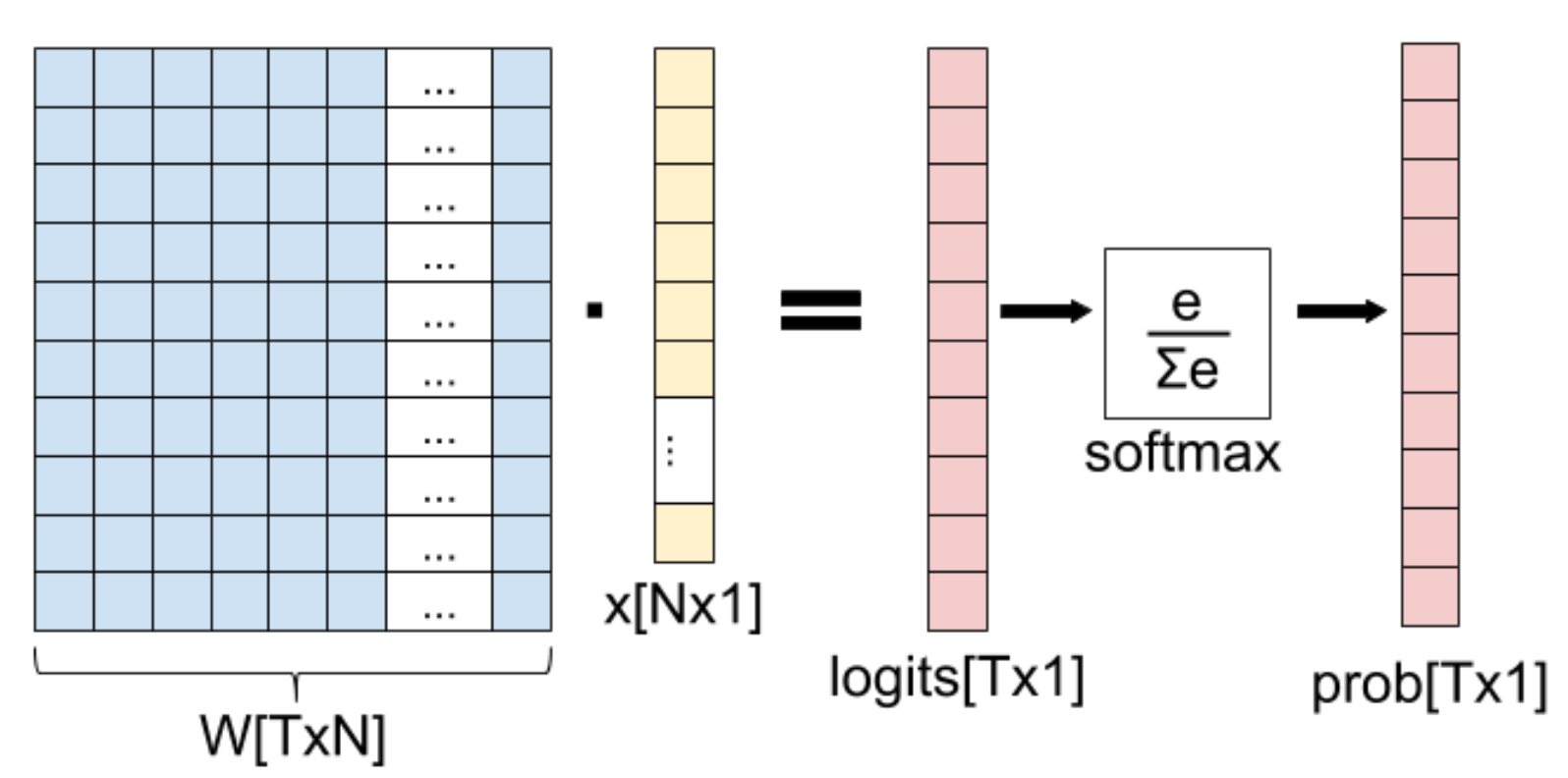# Softmax函数及其导数

## 基础概念

Softmax函数的输入是N维的随机真值向量，输出是另一个N维的真值向量，

S(a):a1a2...aNS1S2...SN

Sj=eajNk=1eakj1...N

## 概率解释

softmax的性质(所有输出的值范围是(0,1)$(0,1)$且和为1.0)使其在机器学习的概率解释中广泛使用。尤其是在多类别分类任务中，我们总是给输出结果对应的类别附上一个概率，即如果我们的输出类别有N种，我们就输出一个N维的概率向量且和为1.0。每一维的值对应一种类别的概率。我们可以将softmax解释如下：

Sj=P(y=j|a)

## 向量计算的准备

Softmax从根本上来说是一种向量函数。它将向量作为输入并输出另一个向量。换言之，它有多个输入和输出，因此我们不能直接就尝试求”softmax的导数”，我们首先要明确：

• 我们想要计算softmax的哪个组成成分(输出的某元素)的导数。
• 由于softmax具有多个输入，所以要计算关于哪个输入元素的偏导数。

Siaj

DS=D1S1D1SN  DNS1DNSN

## softmax的导数

DjSi=Siaj=eaiNk=1eakaj

f(x)=g(x)h(x)h(x)g(x)[h(x)]2

gi=eaihi=k=1Neak

gi$g_i$关于aj$a_j$的导数是eaj$e^{a_j}$当且仅当i=j$i=j$；否则结果为0。

eaiNk=1eakaj=eaieajeai2

eaiNk=1eakaj=eaieajeai2=eaieaj=Si(1Sj)

eaiNk=1eakaj=0eajeai2=eajeai=SjSi

DjSi={Si(1Sj)SjSii=jij

δij={10i=jij

DjSi=Si(δijSj)

• 在雅可比矩阵中使用单位矩阵I$I$来替换δ$\delta$I$I$使用元素的矩阵形式表示了δ$\delta$
• 使用”1”作为函数名而不是克罗内克δ$\delta$，如下所示：DjSi=Si(1(i=j)Sj)$D_jS_i=S_i(1(i=j)-S_j)$。这里1(i=j)意味着当i=j$i=j$时值为1，否则为0。

## 计算softmax和数值稳定性

def softmax(x):
"""Compute the softmax of vector x."""
exps = np.exp(x)
return exps / np.sum(exps)

In : softmax([1, 2, 3])
Out: array([ 0.09003057, 0.24472847,  0.66524096])


In : softmax([1000, 2000, 3000])
Out: array([ nan,  nan,  nan])

Numpy使用的浮点数的数值范围是有限的。对于float64，最大可表示数字的大小为10308$10^{308}$
softmax函数中的求幂运算可以轻松超过这个数字，即使是相当适中的输入。避免这个问题的一个好方法是通过规范输入使其不要太大或者太小，通过观察我们可以使用任意的常量C，如下所示：

Sj=eajNk=1eak=CeajNk=1Ceak

Sj=eaj+log(C)Nk=1eak+log(C)

Sj=eaj+DNk=1eak+D

D也是一个任意常量。对任意D，这个公式等价于前面的式子，这让我们能够更好的进行计算。对于D，一个比较好的选择是所有输入的最大值的负数：
D=max(a1,a2,...,an)

def stablesoftmax(x):
"""Compute the softmax of vector x in a numerically
stable way."""
shiftx = x - np.max(x)
exps = np.exp(shiftx)
return exps / np.sum(exps)

In : stablesoftmax([1000, 2000, 3000])
Out: array([ 0.,  0.,  1.])

## softmax层及其导数

softmax常用于机器学习中，特别是逻辑斯特回归：softmax层，其中我们将softmax应用于全连接层(矩阵乘法)的输出，如图所示。P(W)=S(g(W))=(Sg)(W)

DP(W)=D(Sg)(W)=DS(g(W))Dg(W)

Dg=D1g1D1gT  DNTg1DNTgT

g1=W11x1+W12x2++W1NxN

D1g1=x1D2g1=x2DNg1=xNDN+1g1=0DNTg1=0

Dg=x10x10xN00x10x200xN

Dijgt=(Wt1x1+Wt2x2++WtNxN)Wij={xj0i=tit

DijPt=k=1TDkStDijgk

Dg$Dg$大多数为0，所以最终的结果很简单，仅当i=k$i=k$Dijgk$D_{ij}g_k$不为0；然后它等于xj$x_j$。因此：
DijPt=DiStxj=St(δtiSi)xj

## Softmax和交叉熵损失

xent(p,q)=kp(k)log(q(k))

xent(Y,P)=k=1TY(k)log(P(k))

xent(Y,P)=log(P(y))

y$y$个元素，即Py$P_y$
xent(P)=log(Py)

xent$xent$的雅可比矩阵是1×T$1\times T$的矩阵(一个行向量)。因为输出是一个标量且我们有T$T$个输出(向量P$P$T$T$个元素)：
Dxent(Y,P)=[D1xentD2xentDTxent]

xent(W)=(xentP)(W)=xent(P(W))

Dxent(W)=D(xentP)(W)=Dxent(P(W))DP(W)

y$y$个元素。因此，在雅可比矩阵中，只有Dyxent$D_yxent$是非0的：
Dxent=[00Dyxent0]

iN+j$iN+j$个元素)：
Dijxent(W)=k=1TDkxent(P)DijPk(W)

Dijxent(W)=Dyxent(P)DijPy(W)=1PySy(δyiSi)xj

Dijxent(W)=1SySy(δyiSi)xj=(δyiSi)xj=(Siδyi)xj